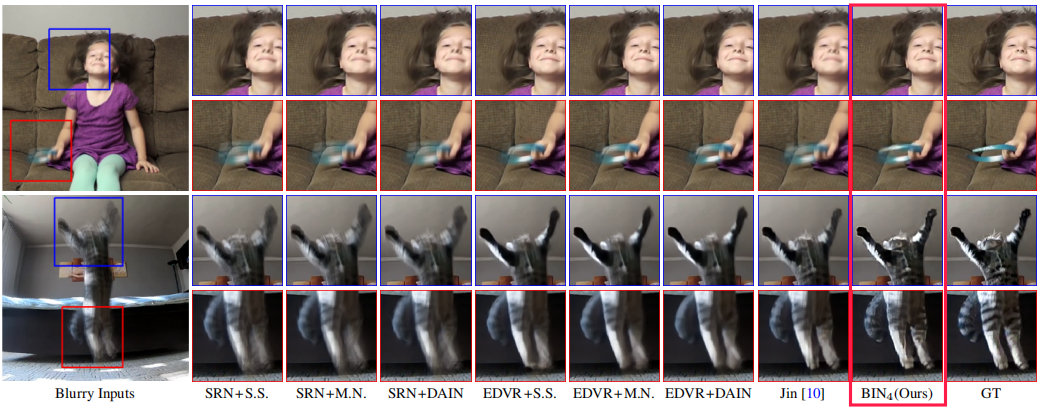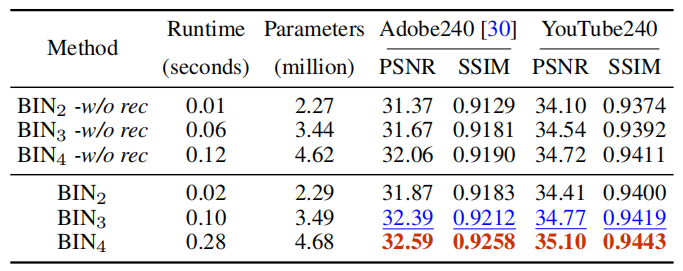# CVPR 2020 三篇有趣的论文解读

## 目录

• Deep Snake for Real-Time Instance Segmentation — 用轮廓做实例分割
• Blurry Video Frame Interpolation — 完美的金字塔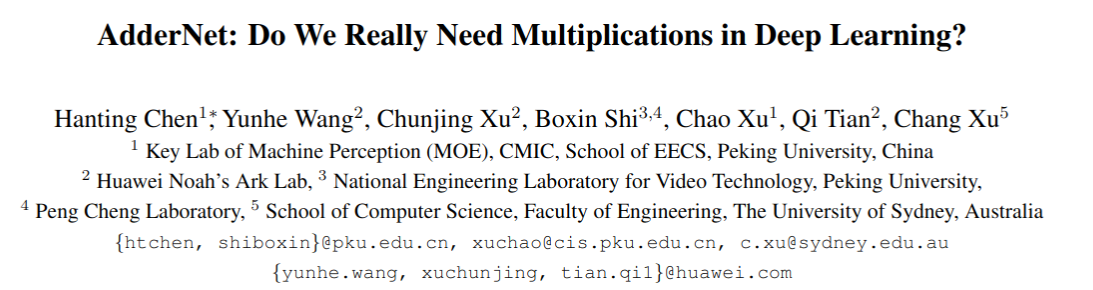[ Y(m, n, t)=sum_{i=0}^{d} sum_{j=0}^{d} sum_{k=0}^{c_{i n}} S(X(m+i, n+j, k), F(i, j, k, t)) ]

[ Y(m, n, t)=-sum_{i=0}^{d} sum_{j=0}^{d} sum_{k=0}^{c_{i n}}|X(m+i, n+j, k)-F(i, j, k, t)| ]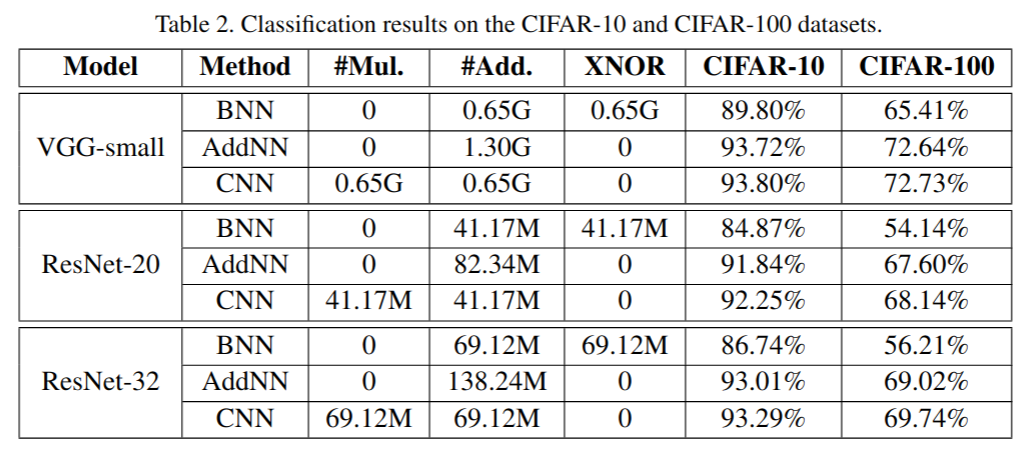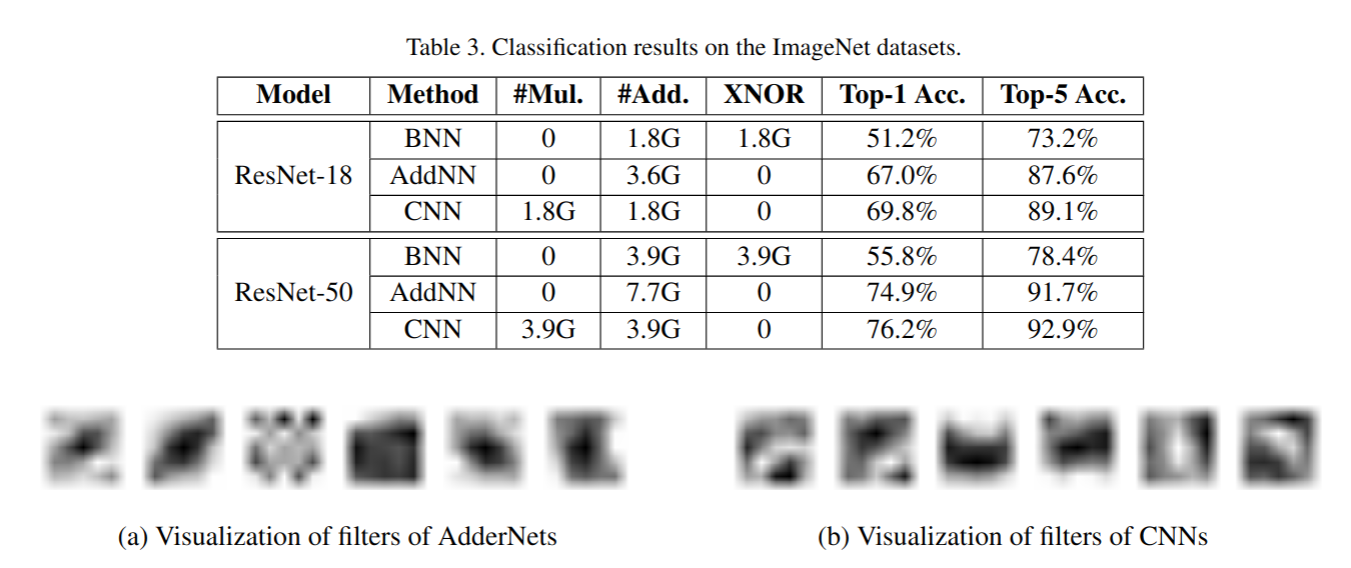## Deep Snake用于实例分割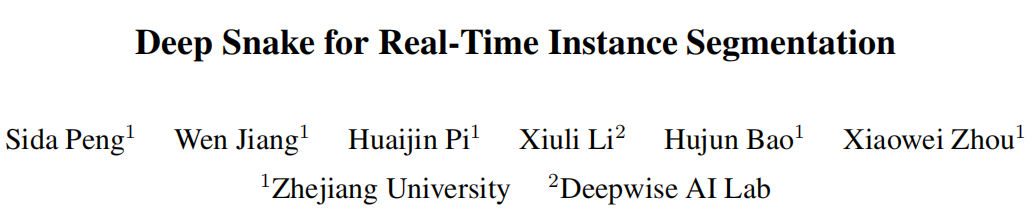repo url：https://github.com/zju3dv/snake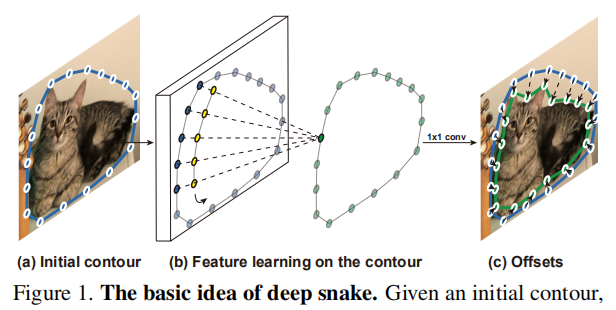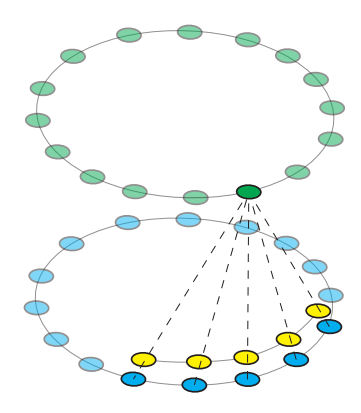[ left(f_{N}right)_{i} triangleq sum_{j=-infty}^{infty} f_{i-j N}=f_{i(bmod N)}\ left(f_{N} * kright)_{i}=sum_{j=-r}^{r}left(f_{N}right)_{i+j} k_{j} ]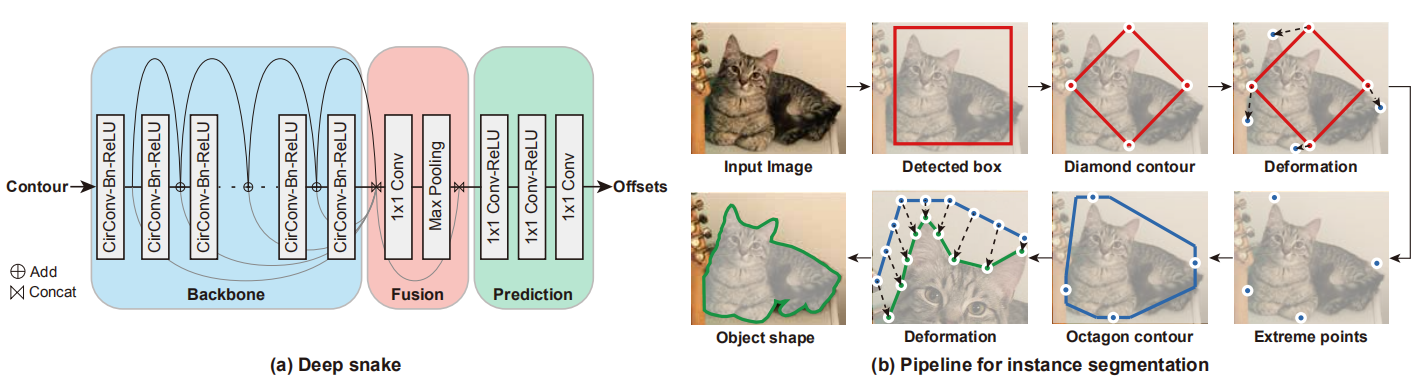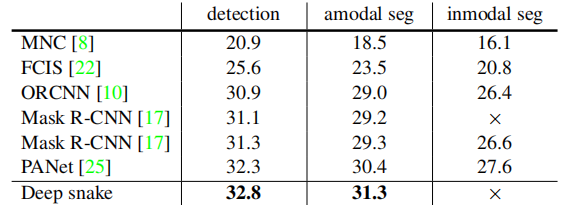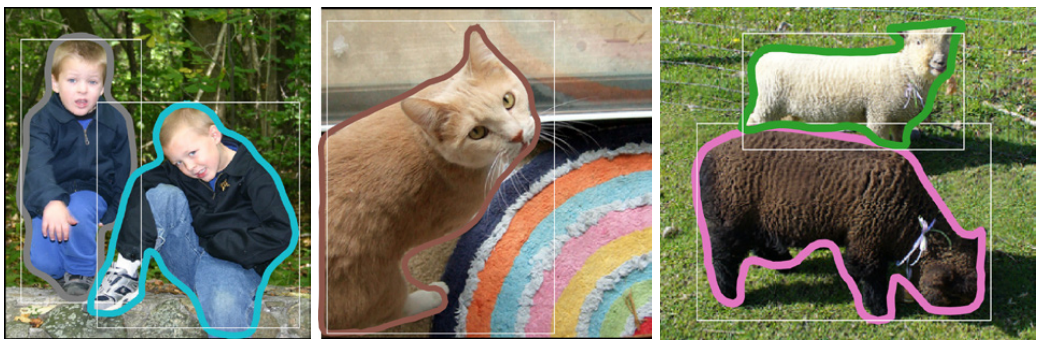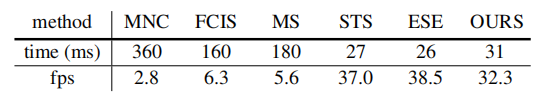## BIN 模糊视频插帧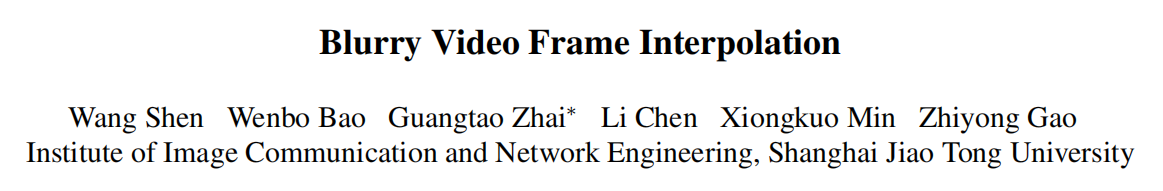repo url : https://github.com/laomao0/BIN

• 1.金字塔模块

• 2.金字塔间的递归模块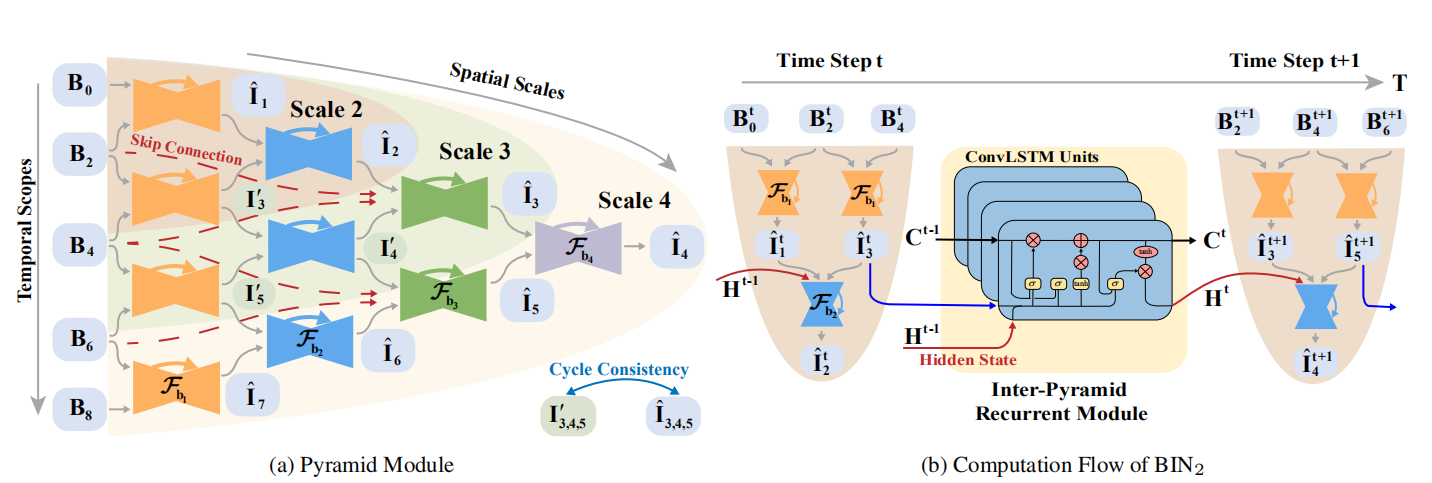[ hat{mathbf{I}}_{1: 1: 2 N-1}=mathcal{F}left(mathbf{B}_{0: 2: 2 N}right) ]

[ hat{mathbf{I}}_{1}=mathcal{F}_{mathrm{b}}left(mathbf{B}_{0}, mathbf{B}_{2}right) ]

[ hat{mathbf{I}}_{1}=mathcal{F}_{mathrm{b_1}}left(mathbf{B}_{0}, mathbf{B}_{2}right)\ {mathbf{I'}}_{3}=mathcal{F}_{mathrm{b_1}}left(mathbf{B}_{2}, mathbf{B}_{4}right)\ hat{mathbf{I}}_{2}=mathcal{F}_{mathrm{b_2}}left(mathbf{hat{I}}_{1}, mathbf{I'}_{3}right)\ hat{mathbf{I}}_{3}=mathcal{F}_{mathrm{b_2}}left(mathbf{hat{I}}_{2}, mathbf{hat{I}}_{4},mathbf{B}_{3},mathbf{B}_{4}right)\ {mathbf{I'}}_{5}=mathcal{F}_{mathrm{b_1}}left(mathbf{B}_{4}, mathbf{B}_{6}right) ]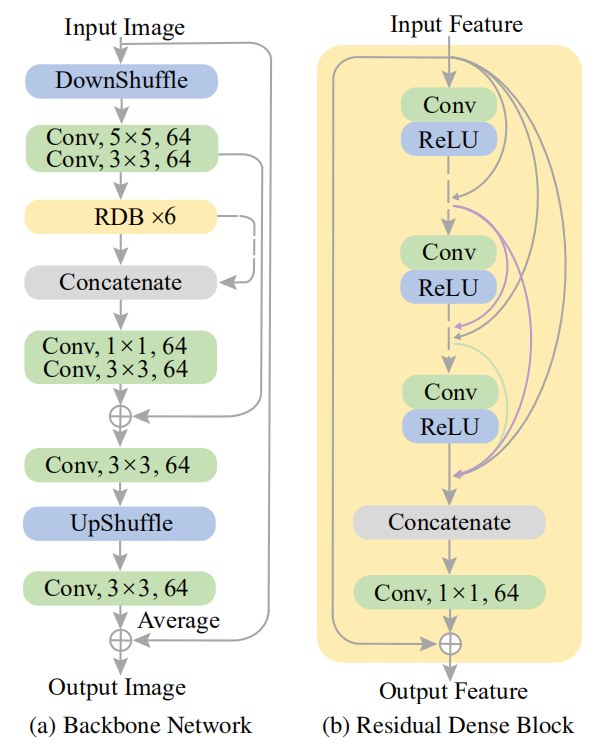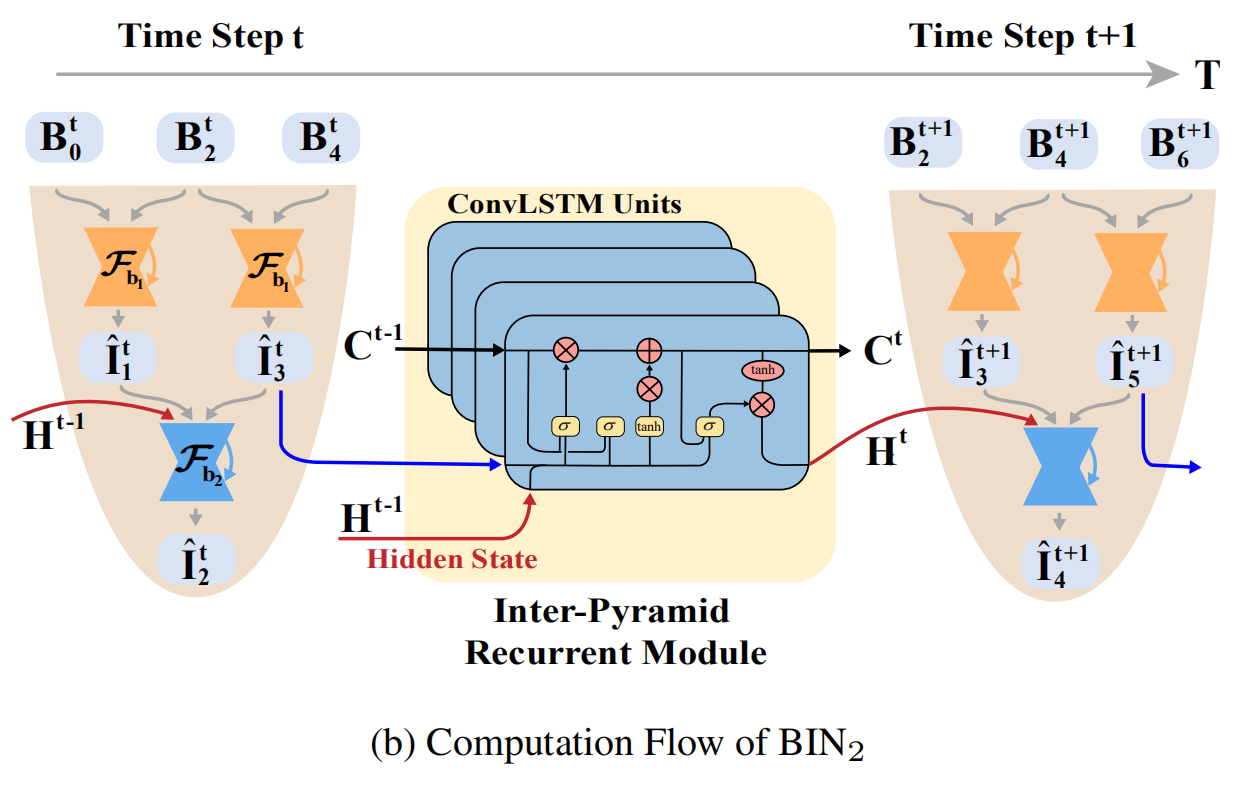ConvLSTM构成的Inter-Pyramid Recurrent Module实际上就是为了传递时空上的信息，这里Time Step为2，(B_2^{t})(B_2^{t+1}) 实际上是同一张输入，但是进入了两个不同的模块，整体step前进了一步，其中的ConvLSTM就是为了传递C和H的，其公式如下：
[ mathbf{H}^{t}, mathbf{C}^{t}=mathcal{F}_{mathrm{c}}left(hat{mathbf{I}}_{3}^{t}, mathbf{H}^{t-1}, mathbf{C}^{t-1}right) ]

[ rho(x)=sqrt{x^{2}+epsilon^{2}}\ mathcal{L}_{p}=frac{1}{T} sum_{t=1}^{T} sum_{n=1}^{2 M-1} rholeft(hat{mathbf{I}}_{n}^{t}-mathbf{G}_{n}^{t}right)\ mathcal{L}_{c}=frac{1}{T} sum_{t=1}^{T} sum_{n in Omega} rholeft(mathbf{I}_{n}^{t}-hat{mathbf{I}}_{n}^{t}right)\ mathcal{L}=mathcal{L}_{p}+mathcal{L}_{c}\ ]